# 二分查找细节详解

the details can be surprisingly tricky… 这句话可以这样理解：思路很简单，细节是魔鬼。

### 二分查找框架

int binarySearch(int[] nums, int target) {
int left = 0, right = ...;

while(...) {
int mid = (right + left) / 2; // int mid = ((right - left) >> 1) + left;
if (nums[mid] == target) {
...
} else if (nums[mid] < target) {
left = ...
} else if (nums[mid] > target) {
right = ...
}
}
return ...;
}


### 寻找一个数（基本的二分搜索）

int binarySearch(int[] nums, int target) {
int left = 0;
int right = nums.length - 1; // 注意

while(left <= right) {
int mid = (right + left) / 2;
if(nums[mid] == target)
return mid;
else if (nums[mid] < target)
left = mid + 1; // 注意
else if (nums[mid] > target)
right = mid - 1; // 注意
}
return -1;
}

1. 为什么 while 循环的条件中是 <=，而不是 < ？
答：因为初始化 right 的赋值是 nums.length-1，即最后一个元素的索引，而不是 nums.length。这二者可能出现在不同功能的二分查找中，区别是：前者相当于两端都闭区间 [left, right]，后者相当于左闭右开区间 [left, right)，因为索引大小为 nums.length 是越界的。
我们这个算法中使用的是前者 [left, right] 两端都闭的区间。这个区间其实就是每次进行搜索的区间，我们不妨称为「搜索区间」
什么时候应该停止搜索呢？当然，找到了目标值的时候可以终止：
if(nums[mid] == target)
return mid;


while(left <= right) 的终止条件是 left == right + 1，写成区间的形式就是 [right + 1, right]，或者带个具体的数字进去 [3, 2]，可见这时候搜索区间为空，因为没有数字既大于等于 33 又小于等于 22 的吧。所以这时候 while 循环终止是正确的，直接返回 -1 即可。
while(left < right) 的终止条件是 left == right，写成区间的形式就是 [left, right]，或者带个具体的数字进去 [2, 2]，这时候搜索区间非空，还有一个数 22，但此时 while 循环终止了。也就是说这区间 [2, 2] 被漏掉了，索引 22 没有被搜索，如果这时候直接返回 -1 就是错误的。

//...
while(left < right) {
// ...
}
return nums[left] == target ? left : -1;

1. 为什么 left = mid + 1，right = mid - 1？我看有的代码是 right = mid或者 left = mid，没有这些加加减减，到底怎么回事，怎么判断？
答：这也是二分查找的一个难点，不过只要你能理解前面的内容，就能够很容易判断。
刚才明确了「搜索区间」这个概念，而且本算法的搜索区间是两端都闭的，即 [left, right]。那么当我们发现索引 mid 不是要找的 target 时，如何确定下一步的搜索区间呢？
当然是 [left, mid - 1] 或者 [mid + 1, right] 对不对？因为 mid 已经搜索过，应该从搜索区间中去除。
2. 此算法有什么缺陷？
答：至此，你应该已经掌握了该算法的所有细节，以及这样处理的原因。但是，这个算法存在局限性。
比如说给你有序数组 nums = [1,2,2,2,3]，target = 2，此算法返回的索引是 22，没错。但是如果我想得到 target 的左侧边界，即索引 11，或者我想得到 target 的右侧边界，即索引 33，这样的话此算法是无法处理的。
这样的需求很常见。你也许会说，找到一个 target，然后向左或向右线性搜索不行吗？可以，但是不好，因为这样难以保证二分查找对数级的复杂度了。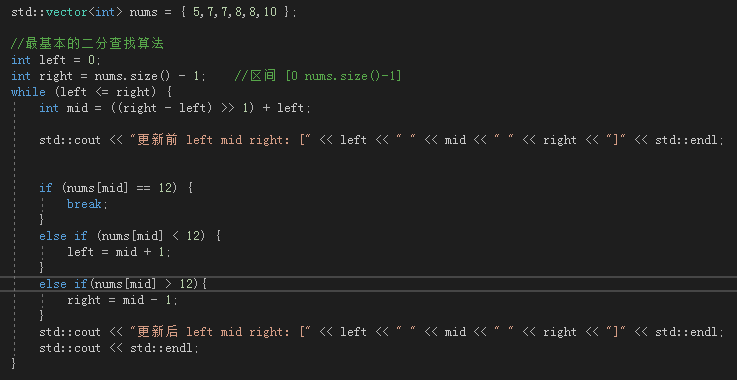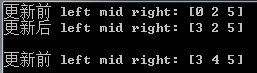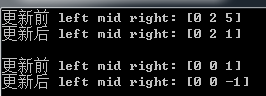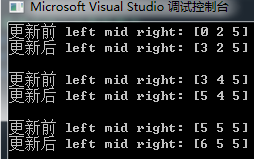while的截止条件是left>right,且如果超过数据的边界，left为-1或者nums.size()。也就是说mid始终在[0-nums.size()-1]之间

### 寻找左侧边界的二分搜索

int left_bound(int[] nums, int target) {
if (nums.length == 0) return -1;
int left = 0;
int right = nums.length; // 注意

while (left < right) { // 注意
int mid = (left + right) / 2;
if (nums[mid] == target) {
right = mid;
} else if (nums[mid] < target) {
left = mid + 1;
} else if (nums[mid] > target) {
right = mid; // 注意
}
}
return left;
}

1. 为什么 while(left < right) 而不是 <= ?
答：用相同的方法分析，因为 right = nums.length 而不是 nums.length - 1。因此每次循环的「搜索区间」是 [left, right) 左闭右开。
while(left < right)终止的条件是 left == right，此时搜索区间 [left, left) 为空，所以可以正确终止。

2. 为什么没有返回 -1 的操作？如果 nums 中不存在 target 这个值，怎么办？
答：因为要一步一步来，先理解一下这个「左侧边界」有什么特殊含义：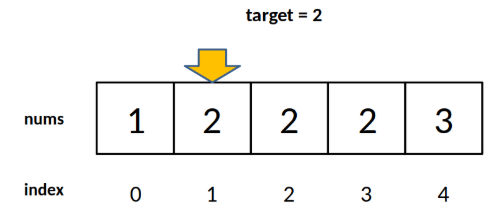对于这个数组，算法会返回 11。这个 11 的含义可以这样解读：nums 中小于 22 的元素有 11 个。
比如对于有序数组 nums = [2,3,5,7], target = 1，算法会返回 0，含义是：nums 中小于 11 的元素有 0 个。
再比如说 nums 不变，target = 8，算法会返回 44，含义是：nums 中小于 88 的元素有 44 个。
综上可以看出，函数的返回值（即 left 变量的值）取值区间是闭区间 [0, nums.length]，所以我们简单添加两行代码就能在正确的时候 return -1：
while (left < right) {
//…
}
// target 比所有数都大
if (left == nums.length) return -1;
// 类似之前算法的处理方式
return nums[left] == target ? left : -1;

3. 为什么 left = mid + 1，right = mid ？和之前的算法不一样？
答：这个很好解释，因为我们的「搜索区间」是 [left, right) 左闭右开，所以当 nums[mid] 被检测之后，下一步的搜索区间应该去掉 mid 分割成两个区间，即 [left, mid) 或 [mid + 1, right)。

4. 为什么该算法能够搜索左侧边界？
答：关键在于对于 nums[mid] == target 这种情况的处理：
if (nums[mid] == target)
right = mid;
可见，找到 target 时不要立即返回，而是缩小「搜索区间」的上界 right，在区间 [left, mid)中继续搜索，即不断向左收缩，达到锁定左侧边界的目的。

5. 为什么返回 left 而不是 right？
答：都是一样的，因为 while 终止的条件是 left == right。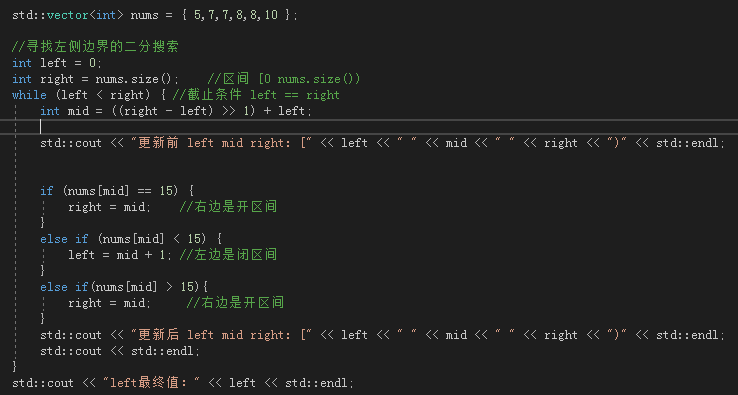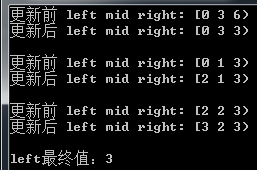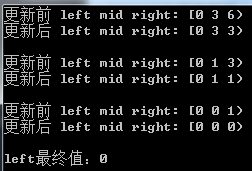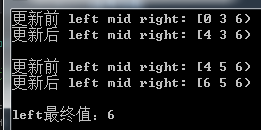while的截止条件是leftright,且如果超过数据的边界，left为0或者nums.size()。

nums.size()，则说明没有找到左边界。去除该条件left的范围[0 nums.szie()-1]，用nums[left]==target来确保是否找到target值。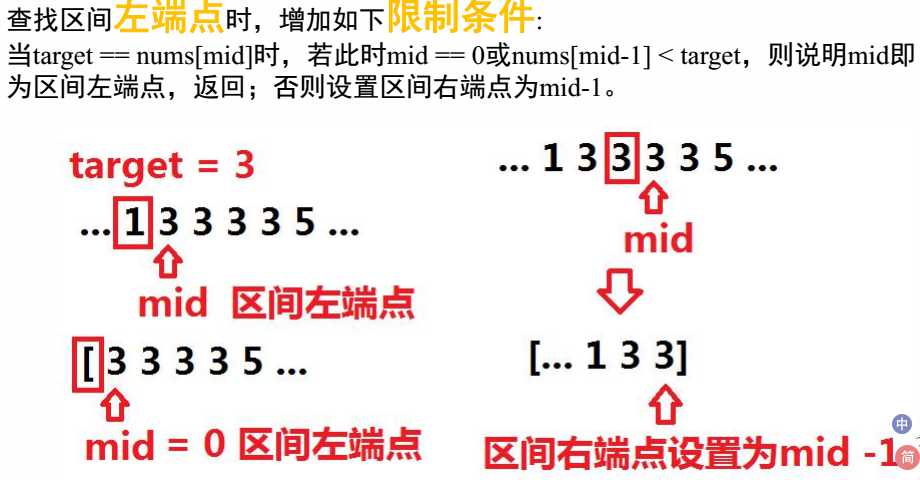int begin = 0;
int end = nums.size() - 1;
while(begin <= end){
int mid = ((end-begin)>>1) + begin;
if(nums[mid] == target){
if(mid == 0 || nums[mid - 1] < target){
return mid;
}
end = mid - 1;
}else if(nums[mid] < target){
begin = mid + 1;
}else if(nums[mid] > target){
end = mid - 1;
}
}


### 寻找右侧边界的二分查找

int right_bound(int[] nums, int target) {
if (nums.length == 0) return -1;
int left = 0, right = nums.length;

while (left < right) {
int mid = (left + right) / 2;
if (nums[mid] == target) {
left = mid + 1; // 注意
} else if (nums[mid] < target) {
left = mid + 1;
} else if (nums[mid] > target) {
right = mid;
}
}
return left - 1; // 注意
}

1. 为什么这个算法能够找到右侧边界？
答：类似地，关键点还是这里：
if (nums[mid] == target) {
left = mid + 1;


2. 为什么最后返回 left - 1 而不像左侧边界的函数，返回 left？而且我觉得这里既然是搜索右侧边界，应该返回 right 才对。

if (nums[mid] == target) {
left = mid + 1;
// 这样想: mid = left - 1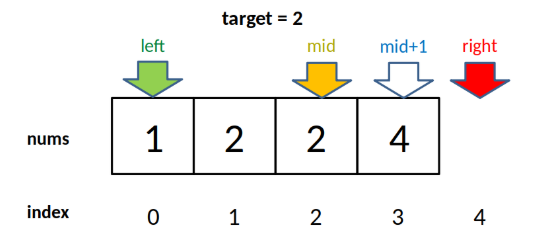1. 为什么没有返回 -1−1 的操作？如果 nums 中不存在 target 这个值，怎么办？
答：类似之前的左侧边界搜索，因为 while 的终止条件是 left == right，就是说 left 的取值范围是 [0, nums.length]，所以可以添加两行代码，正确地返回 -1−1：
while (left < right) {
// ...
}
if (left == 0) return -1;
return nums[left-1] == target ? (left-1) : -1;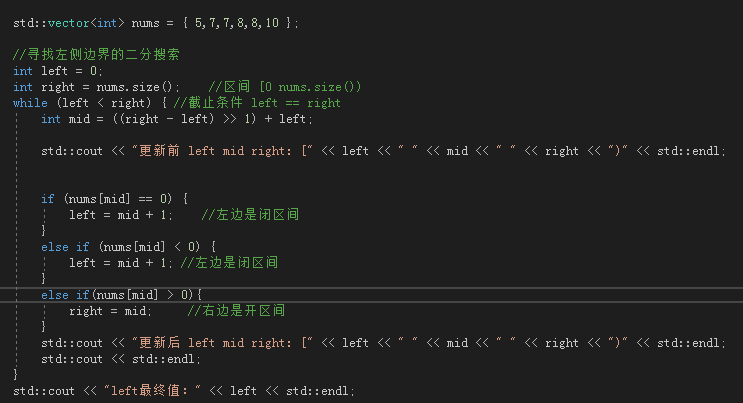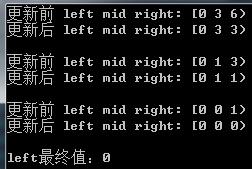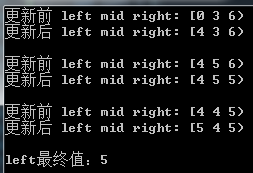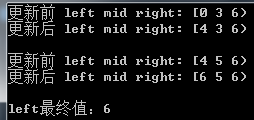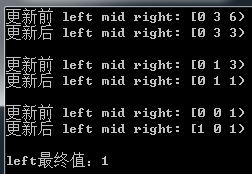if (left == 0) return -1;
return nums[left-1] == target ? (left-1) : -1;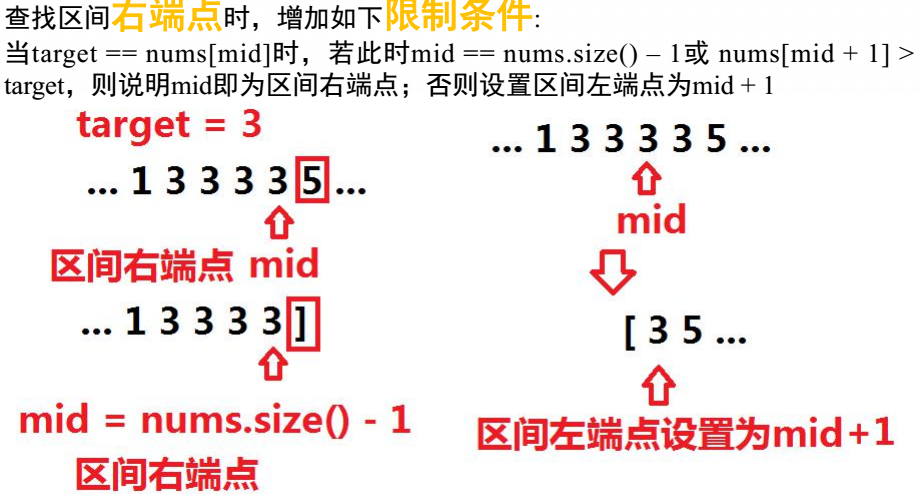int begin = 0;
int end = nums.size() - 1;
while(begin <= end){
int mid = ((end-begin)>>1) + begin;
if(nums[mid] == target){
if(mid == (nums.size() - 1) || target < nums[mid + 1]){
return(mid);
}
begin = mid + 1;
}else if(nums[mid] < target){
begin = mid + 1;
}else if(nums[mid] > target){
end = mid - 1;
}
}


### 总结

leetcode 34题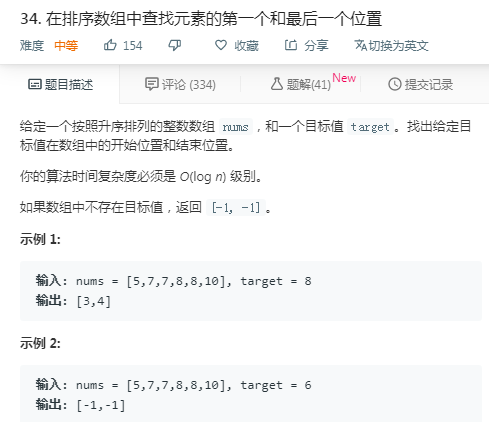class Solution {
public:
vector<int> searchRange(vector<int>& nums, int target) {

vector<int> result;
int begin = 0;
int end = nums.size(); // [0 nums.size()) 右开区间，注意检查begin==end的情况
//找左边
while(begin < end){
int mid = ((end-begin)>>1) + begin;
if(nums[mid] == target){
end = mid;
}else if(nums[mid] > target){
end = mid;
}else if(nums[mid] < target){
begin = mid + 1;
}
}

//begin == end  可能的取值为0、nums.length或者(0-nums.length)
// target比所有数都大,也就是left==nums.length
if (begin == nums.size()) return {-1,-1};
// target比所有数都小，这时begin为0；begin处理[0 - nums.length-1]都有可能等于target
if(nums[begin] == target){
result.push_back(begin);
}else{ //这时begin为0，且target比所有数都小，即没有找到目标值
return {-1,-1};
}

//找右边
begin = 0;
end = nums.size();
while(begin<end){
int mid = ((end-begin)>>1) + begin;
if(nums[mid] == target){
begin = mid + 1; //闭区间
}else if(nums[mid] > target){
end = mid;       //开区间
}else if(nums[mid] < target){
begin = mid + 1;
}
}

//正常处理左边
//begin == end  可能的取值为0、nums.length或者(0-nums.length)
//if(left == 0) return {-1,-1};
//if(nums[left-1]==target){  //left可能为[1-nums.length] left-1可能为[0-nums.length-1]
//result.push_back(begin-1);
//}else{
//return {-1,-1};
//}

//由于找左边时，已经确保数组中有target，这里只需要返回，不需要特殊处理
result.push_back(begin-1);
return result;
}
};

10-09140
08-02103003-08124
10-12239